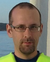# Suggest an Idea

Announcements
Skip the ticket, Chat with Qlik Support instead for instant assistance.

# Allow the user to sort the XIRR() function by datesContributor II

## Allow the user to sort the XIRR() function by dates

This development would resolve the issue about the XIRR calculation being incorrect in most cases.

Today, the XIRR() function has 3 main limitations:

1. The values array must contain at least one positive value and one negative value.
2. All Dates must be valid interpretations of dates that correspond to values.
3. Values and dates must be in chronological order.

The points 1 & 2 are logical and can be corrected in the script if there is any issue.
The problem comes from the point 3, as it is not possible for now to order the data directly in the script.
Therefore, the XIRR() function returns, most of the time, incorrect results.

To work correctly, we should add in the definition of the XIRR() function, a new parameter being a column to determine the order of the function processing.
Let's say that my table is composed of 2 columns (Paiement & DateOfPaiement), the function would then look like XIRR(Paiement, DateOfPaiement, DateOfPaiement).

Tags (3)
1 CommentEmployee

Hi,

There is a misunderstanding I want to clear out. The order of the values and dates actually does not have to be in chronologically order for the Qlik XIRR function. And in fact, changing the order of the data should not change the result of XIRR function. For XNPV function on the other hand, the order matters because the first date found is used to set the “present day” date used in the calculation. Although XIRR makes use of XNPV internally, it does so by the equation XNPV=0 and for this special case of XNPV the order of the data does not matter.

From what I understand, the XIRR sorting issue hypothesis was brought forward in this community article (I have posted an explanation there):

https://community.qlik.com/t5/App-Development/XIRR-Value-Sorting-Issue/td-p/1742977

In short, Excel XIRR failed for some inputs and returned zero (or close to zero). This was then incorrectly believed to be the correct answer. But after resorting the same data Excel was suddenly able to do the XIRR calculation and returned the actual correct answer, which is the same answer as Qlik XIRR is returning. This was probably misunderstood as the sort order should affect the return value of XIRR.I have responded in that thread and explained why sort order should not matter for XIRR.

However, there was a separate issue in Qlik XIRR which caused problems in a less common use cases. This issue has now been fixed in QCS, it is explained in this article:

https://community.qlik.com/t5/Member-Articles/XIRR-function-Fix-and-Update/ta-p/2049021

Subscribe by Topic